Latest Banking jobs   »

# Quantitative Aptitude Quiz For RBI Grade B Phase 1 2023 -02nd June

Directions (1-5): Study the bar chart given below and answer the following questions.
Bar chart shows the cost price of 5 different articles (A, B, C, D & E) and amount of discount allowed on these 5 articles.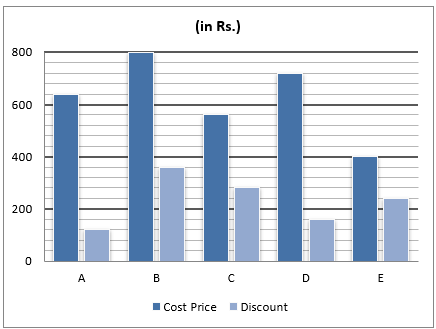Q1. The selling price of A is Rs.260 more than that of C and selling price of C is 50% more than discount allowed on C. Find marked price of A & C together is what percent of cost price of B & E together.
(a) 75%
(b) 125%
(c) 175%
(d) 100%
(e) 150%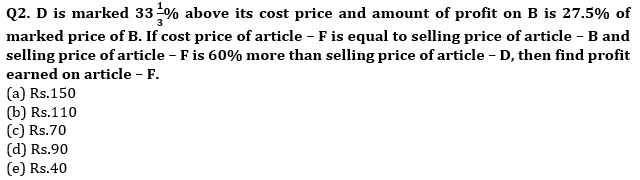Q3. The ratio of marked price of E to profit earned on E is 25 : 9 and marked price of C is equal to cost price of B. Find selling price of C & E together are how much more or less than cost price of A & B together?
(a) Rs.200
(b) Rs.120
(c) Rs.40
(d) Rs.160
(e) Rs.80

Q4. The marked price of A & B together is Rs.2000 and ratio of selling price of A to that of B is 17 : 21. Find amount of profit/loss earned by a person, if he sold 12 units of article – A and 17 units of article – B.
(a) Rs.1160
(b) Rs.1540
(c) Rs.1820
(d) Rs.820
(e) Rs.640

Q5. The profit earned on B is equal to that earned on E and selling price of B is equal to marked price of D. If selling price of D is 84% of its marked price, then find marked price of B & E together.
(a) Rs.2340
(b) Rs.2280
(c) Rs.2200
(d) Rs.2480
(e) Rs.2400

Directions (6 – 10): Bar graph given below shows percentage of students passed out of total students in four different schools and percentage of girls passed out of total passed students in these four different schools in annual exam. Read the data carefully and answer the questions.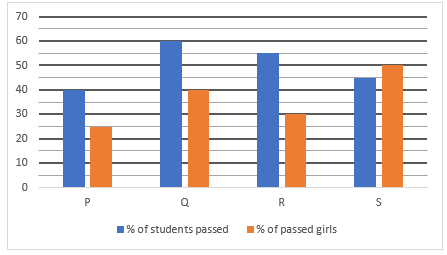Q6. The total boys passed from P is 900 and total girls failed from Q is 640. If total girls failed from Q is 36% less than of total students failed from Q, then find ratio of total students participated in exam from Q to that of from P?
(a) 5 : 9
(b) 5 : 7
(c) 3 : 5
(d) 5 : 6
(e) 4 : 7

Q7. Total boys failed from school Q is 60% of total students failed from that school and total boy passed from same school is 1440. Total failed boys from R is 192 more than total female passed from same school. Find difference between total girls failed from Q & R, if boys failed from R is 42% less than total students failed from R.
(a) 272
(b) 242
(c) 252
(d) 240
(e) 262

Q8. If total number of girls passed from S is 1125 and total failed boys are 133 1/3% more than that of total failed girls from same school, then find the difference between number of failed boys and failed girls from school S?
(a) 1100
(b) 1000
(c) 1110
(d) 900
(e) 1200

Q9. If difference between passed boys and passed girls from P is 600 and total boys passed from S is 1350, then find total failed students from S is what percent more than total students participated from school P?
(a) 15%
(b) 10%
(c) 12%
(d) 5%
(e) 20%

Q10. If total students participated in exam from school R is 50% more than that of from P and difference between passed boys from both the school is 2220, then find the average number of girls passed from both the schools?
(a) 1100
(b) 1000
(c) 1390
(d) 900
(e) 1200

Solutions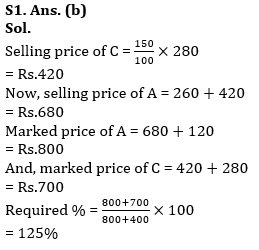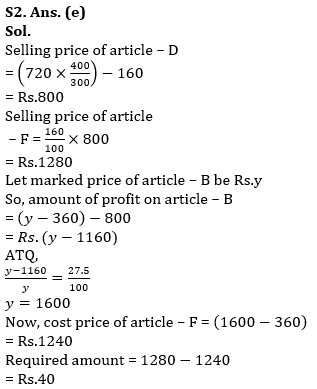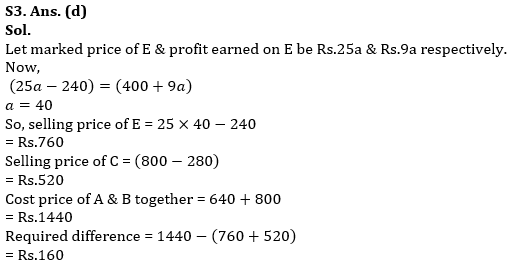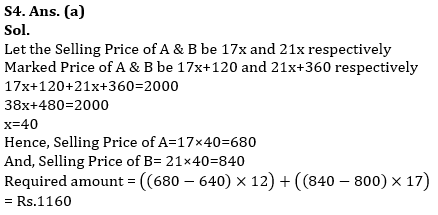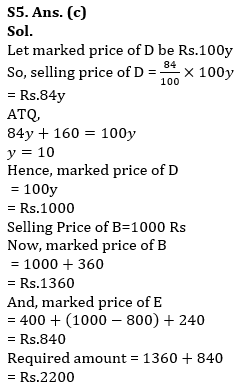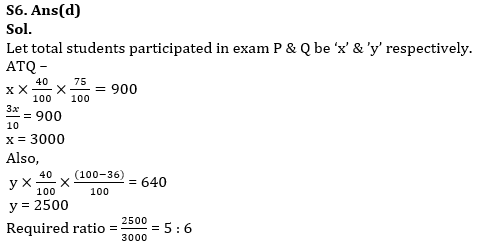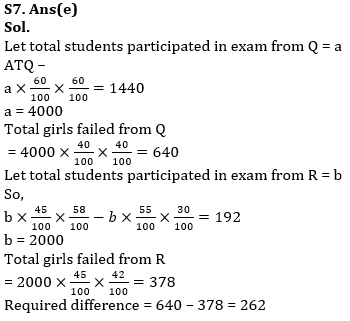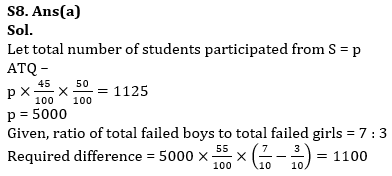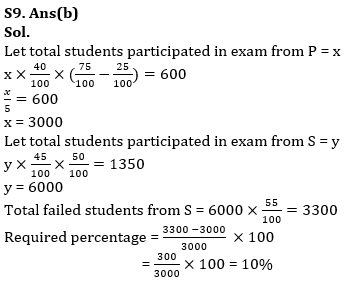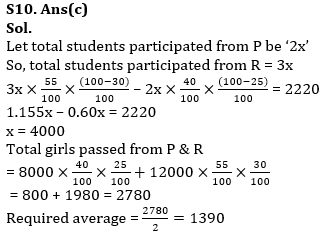## FAQs

### How many sections are there in the RBI Grade B Phase 1 Exam?

There are 4 sections in the RBI Grade B Phase 1 Exam i.e. English Language, General Awareness, Quantitative Aptitude & Reasoning.

#### Congratulations!Union Budget 2023-24: Free PDF